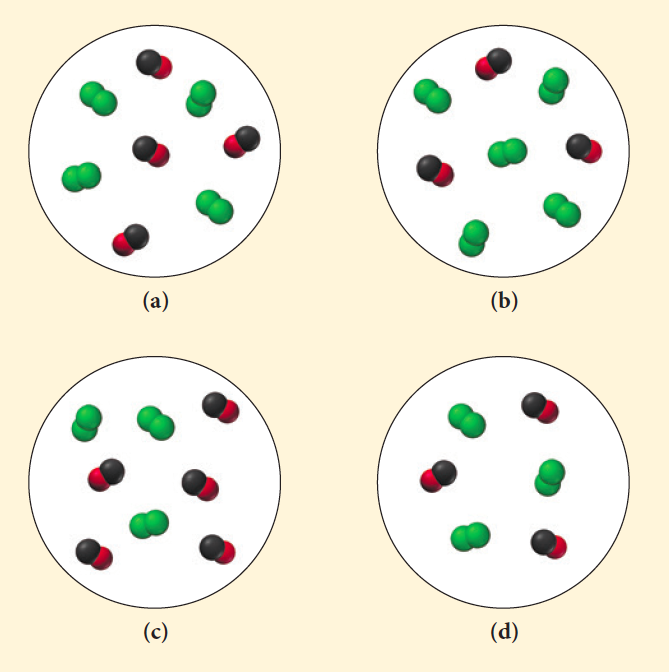# Problem: Carbon monoxide and chlorine gas react to form phosgene (COCl2) according to the equation: CO(g) + Cl2(g) → COCl2(g). The rate law for the reaction is Rate = k[Cl2]3/2[CO]. Which representation of the mixture of chlorine gas and carbon monoxide gas has the fastest initial rate?

###### FREE Expert Solution

Highest initial rate

• highest [Cl2]

(a) Rate = k3/2 = 32

91% (200 ratings)###### Problem Details

Carbon monoxide and chlorine gas react to form phosgene (COCl2) according to the equation: CO(g) + Cl2(g) → COCl2(g). The rate law for the reaction is Rate = k[Cl2]3/2[CO]. Which representation of the mixture of chlorine gas and carbon monoxide gas has the fastest initial rate?Frequently Asked Questions

What scientific concept do you need to know in order to solve this problem?

Our tutors have indicated that to solve this problem you will need to apply the Rate Law concept. You can view video lessons to learn Rate Law. Or if you need more Rate Law practice, you can also practice Rate Law practice problems.

What professor is this problem relevant for?

Based on our data, we think this problem is relevant for Professor Russell's class at UTAH.